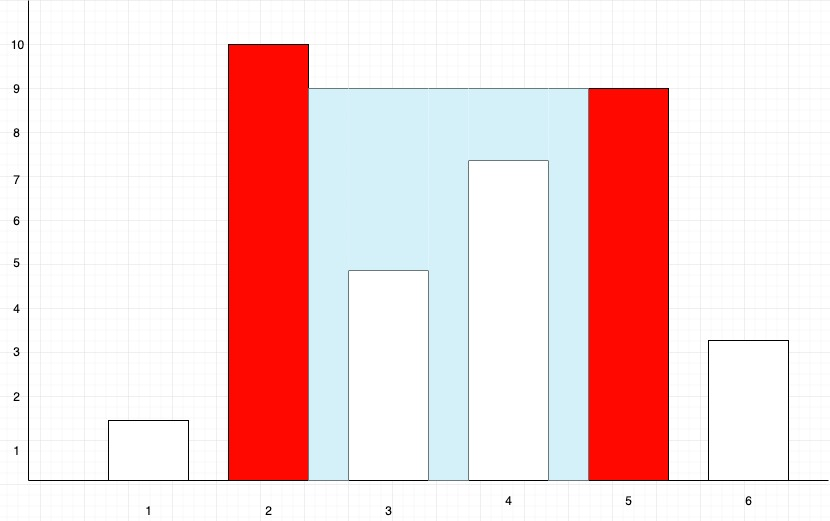Problem title
Difficulty
Avg time to solve

Complete String
Hard
40 mins
Check Palindrome
Easy
--
Encode the Message
Easy
18 mins
Sudoku Solver
Hard
25 mins
Word Break-1
Moderate
36 mins
Game of 3
Easy
30 mins
Colorful Knapsack
Hard
45 mins
Longest Increasing Subsequence
Moderate
30 mins
Longest Palindromic Substring
Easy
20 mins
Top View Of Binary Tree
Moderate
25 mins35

# Container With Most Water

Difficulty: MEDIUM
Avg. time to solve
15 min
Success Rate
90%

Problem Statement

#### Given a sequence of ‘N’ space-separated non-negative integers A,A,A,......A[i]…...A[n]. Where each number of the sequence represents the height of the line drawn at point 'i'. Hence on the cartesian plane, each line is drawn from coordinate ('i',0) to coordinate ('i', 'A[i]'), here ‘i’ ranges from 1 to ‘N’. Find two lines, which, together with the x-axis forms a container, such that the container contains the most area of water.

##### Note :
``````1. You can not slant the container i.e. the height of the water is equal to the minimum height of the two lines which define the container.

2. Do not print anything, you just need to return the area of the container with maximum water.
``````
##### Example``````For the above Diagram, the first red marked line is formed between coordinates (2,0) and (2,10), and the second red-marked line is formed between coordinates (5,0) and (5,9). The area of water contained between these two lines is (height* width) = (5-2)* 9 = 27, which is the maximum area contained between any two lines present on the plane. So in this case, we will return 3* 9=27.
``````
##### Input format :
``````The first line of input contains an integer ‘T’ denoting the number of test cases.

The next ‘2*T’ lines represent the ‘T’ test cases.

The first line of each test case contains the number of elements in the sequence.

The second line of each test case contains ‘n’ space-separated integers which is the given sequence.
``````
##### Output format :
``````For every test case return the area of the container which can hold the maximum amount of water using any pair of lines from the given sequence.
``````
##### Constraints:
``````1 <= T <= 50
0 <= N <= 10^4
1 <= A[i] <= 10^5

Time Limit: 1 sec
``````
##### Sample Input 1 :
``````2
5
4 3 2 1 4
3
1 2 1
``````
##### Sample Output 1 :
``````16
2
``````
##### Explanation of The Sample Input 1:
``````For the first case:
We can create ‘n(n+1)/2’ different containers using ‘N' containers for example with 1st and 3rd line we can create a container of area = (3-1)*min(4,2)=4.

All Possible Containers:

Lines used          Area
4,3         area=min(4,3)*1=3
4,2         area=min(4,2)*2=4
4,1         area=min(4,1)*3=3
4,4         area=min(4,4)*4=16
3,2         area=min(3,2)*1=2
3,1         area=min(3,1)*2=2
3,4         area=min(3,4)*3=9
2,1         area=min(2,1)*1=1
2,4         area=min(2,4)*2=4
1,4         area=min(1,4)*1=1

But among all such containers the one with the maximum area will be formed by using the first and last line, the area of which is : (5-1)*min(4,4)=16.

Hence we return 16.

For the second case:
We can take the first and third line to get an area of (3-1)*min(1,1)=2 which is the maximum possible area in this sequence.
``````
##### Sample Input 2 :
``````2
5
12 4 6 8 1
3
1 2 3
``````
##### Sample Output 2 :
``````24
2
``````Console